# RS Aggarwal Solutions for Class 6 Maths Chapter 12 Parallel Lines

Given here RS Aggarwal Solutions for Class 6 Maths Chapter 12 Parallel Lines. Students can refer RS Aggarwal Solutions in PDF format and practice them thoroughly to excel with good marks in the academic year. By going through RS Aggarwal Solutions in depth students clear their doubts and gain confidence in solving them. Practicing textbook questions will help the students to understand the chapter effectively.

BYJU’S experts have solved the RS Aggarwal Solutions in PDF format for students, which help them to understand the chapter without any obstacles. Download PDF of Class 6 Chapter 12 in their respective links.

## Download PDF of RS Aggarwal Solutions for Class 6 Maths Chapter 12 Parallel Lines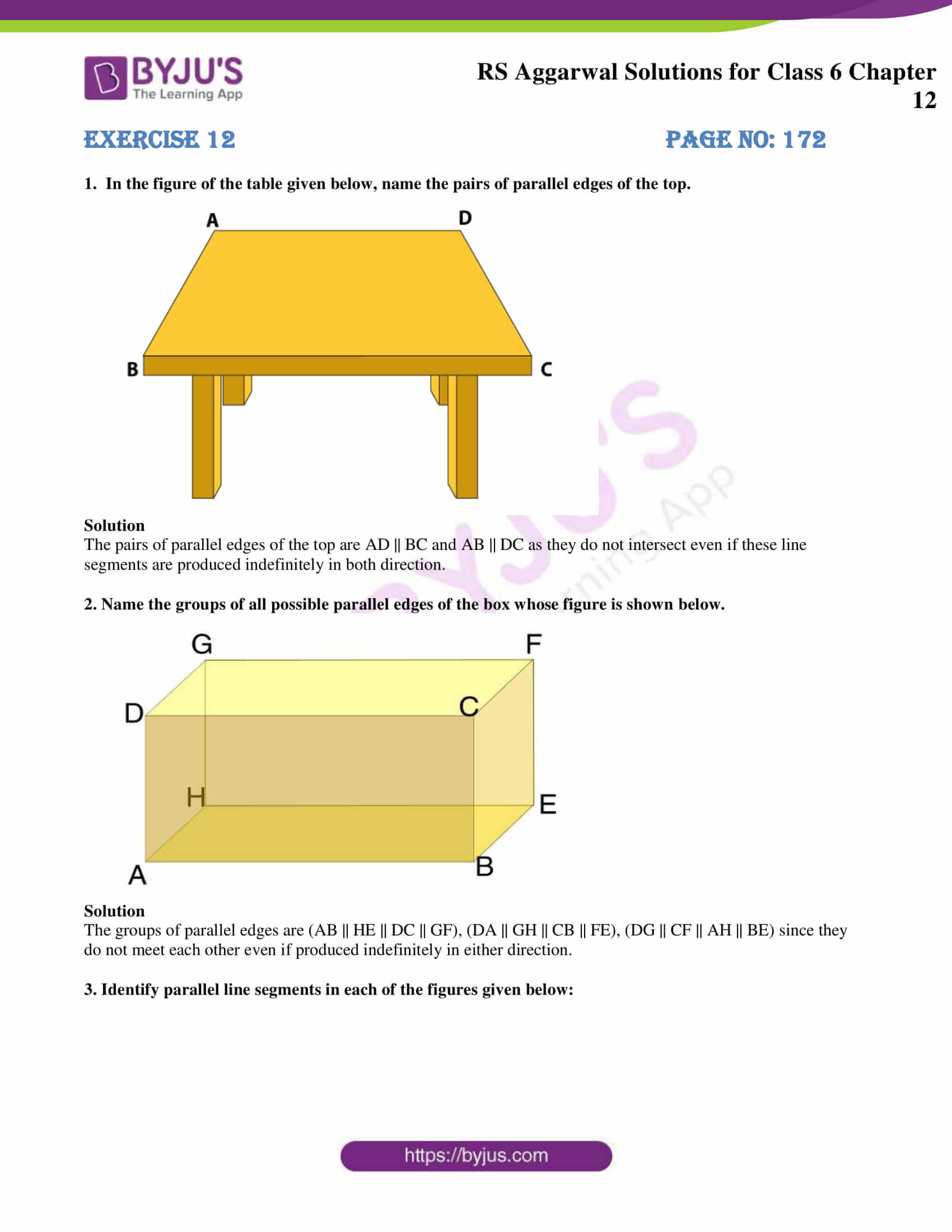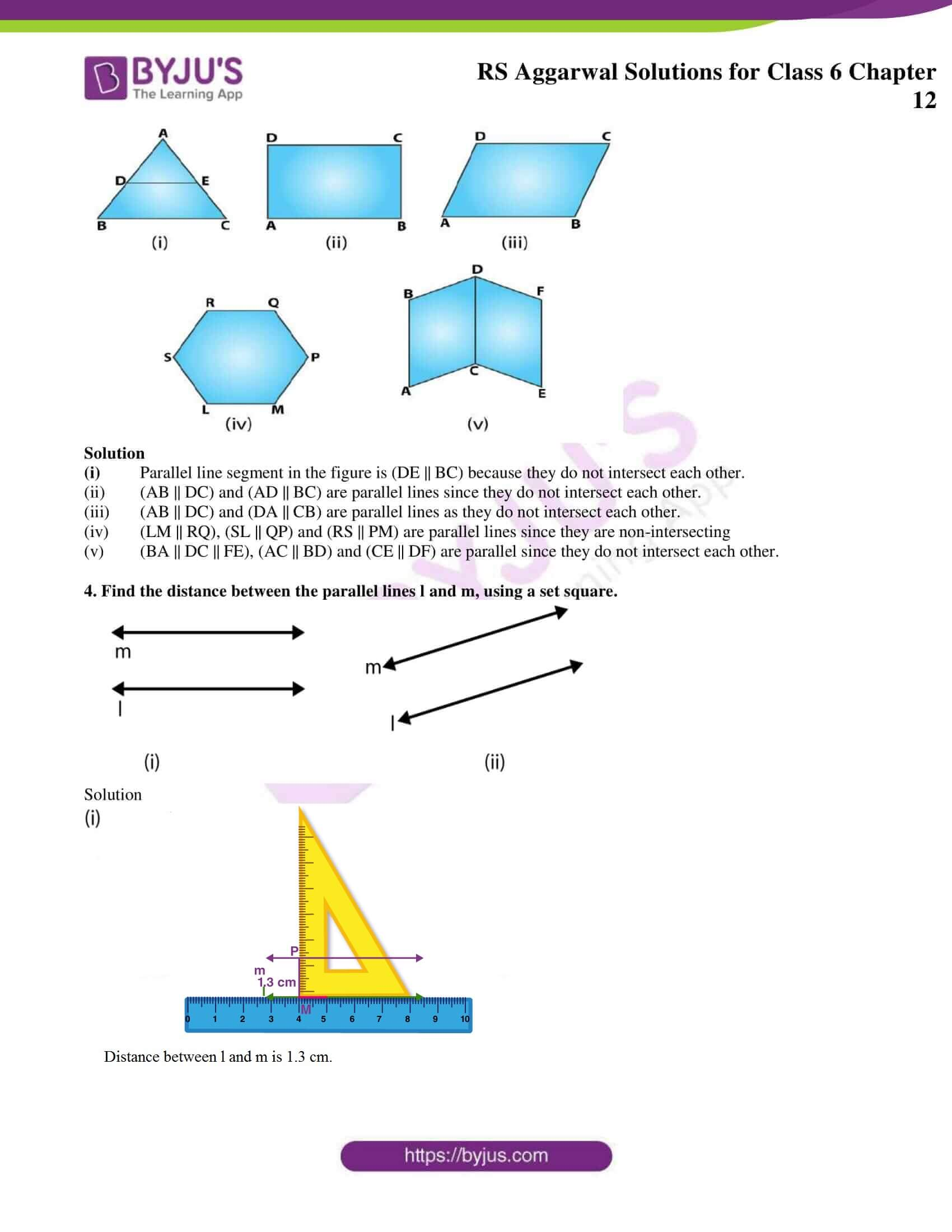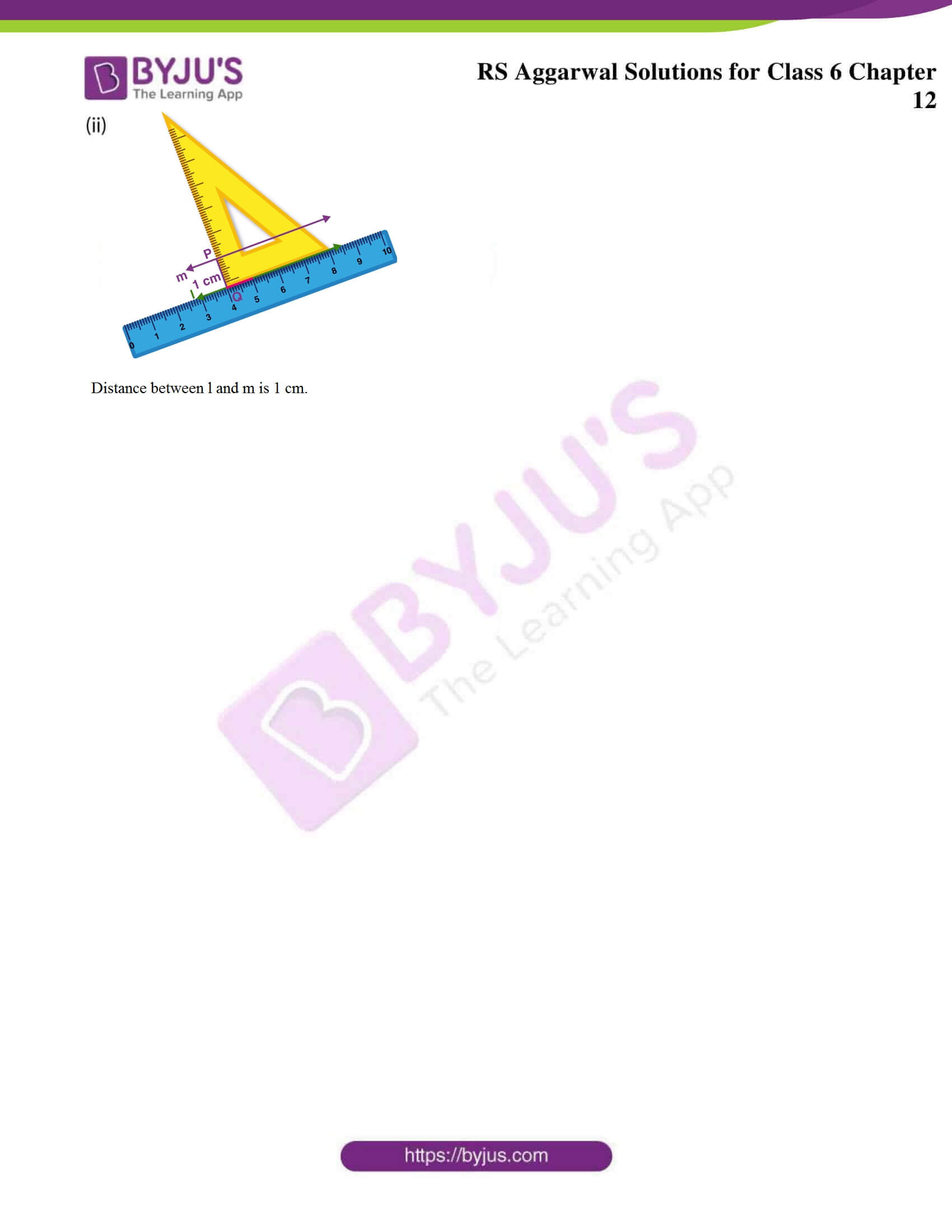## Exercise 12 PAGE NO: 172

1. In the figure of the table given below, name the pairs of parallel edges of the top.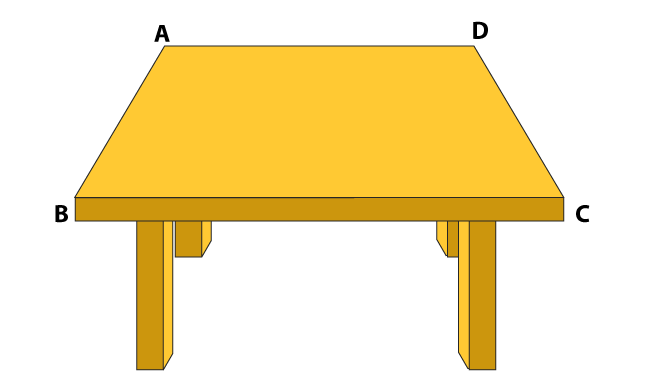Solution

The pairs of parallel edges of the top are AD || BC and AB || DC as they do not intersect even if these line segments are produced indefinitely in both direction.

2. Name the groups of all possible parallel edges of the box whose figure is shown below.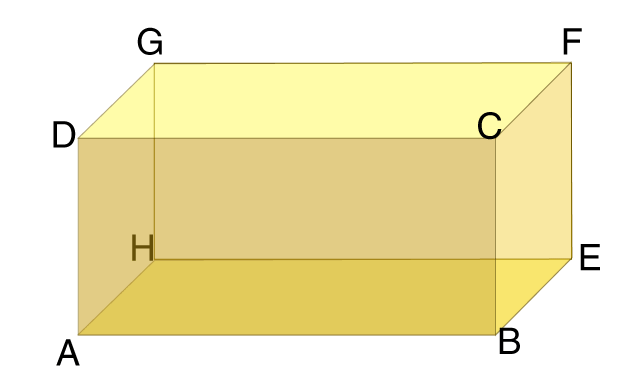Solution

The groups of parallel edges are (AB || HE || DC || GF), (DA || GH || CB || FE), (DG || CF || AH || BE) since they do not meet each other even if produced indefinitely in either direction.

3. Identify parallel line segments in each of the figures given below: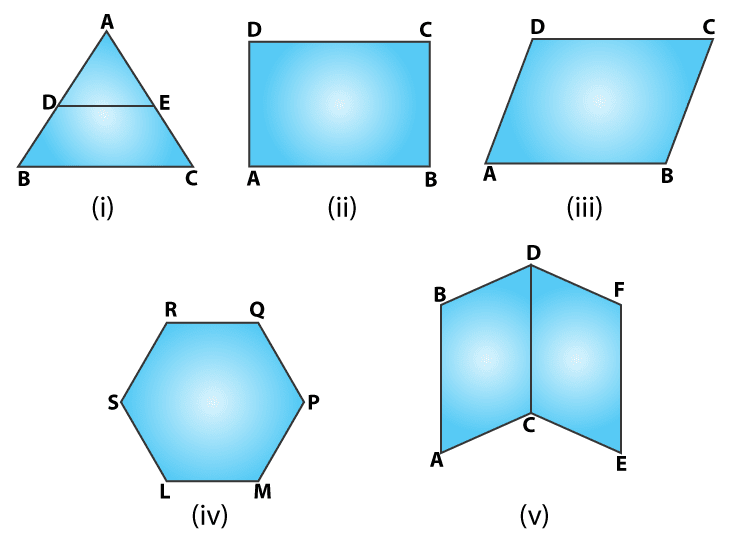Solution

(i) Parallel line segment in the figure is (DE || BC) because they do not intersect each other.

(ii) (AB || DC) and (AD || BC) are parallel lines since they do not intersect each other.

(iii) (AB || DC) and (DA || CB) are parallel lines as they do not intersect each other.

(iv) (LM || RQ), (SL || QP) and (RS || PM) are parallel lines since they are non-intersecting

(v) (BA || DC || FE), (AC || BD) and (CE || DF) are parallel since they do not intersect each other.

4. Find the distance between the parallel lines l and m, using a set square.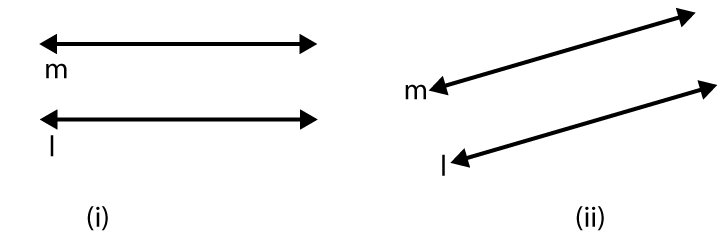Solution

(i)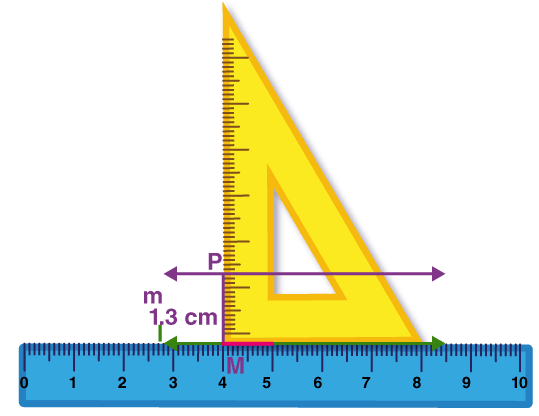Distance between l and m is 1.3 cm

(ii)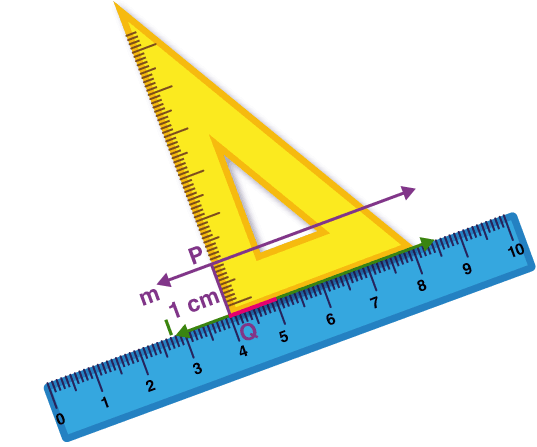Distance between l and m is 1 cm

### RS Aggarwal Solutions for Class 6 Maths Chapter 12 Parallel Lines

Chapter 12 – Parallel Lines includes 1 exercise. For each question, RS Aggarwal Solutions of Class 6 have been given in detail. Let’s have a glance at the topics mentioned in this chapter.

• Parallel Lines
• Distance between two parallel lines
• Parallel segments and parallel rays
• How to test whether given lines are parallel

Also, access RS Aggarwal Solutions for Class 6 Chapter 12

Exercise 12

### Chapter Brief of RS Aggarwal Solutions for Class 6 Maths Chapter 12 – Parallel Lines

Two lines in a plane which do not meet even after extending indefinitely in either direction, are known as parallel lines. Examples of parallel lines are opposite edges of a blackboard, the opposite edges of a ruler, railway lines, etc.